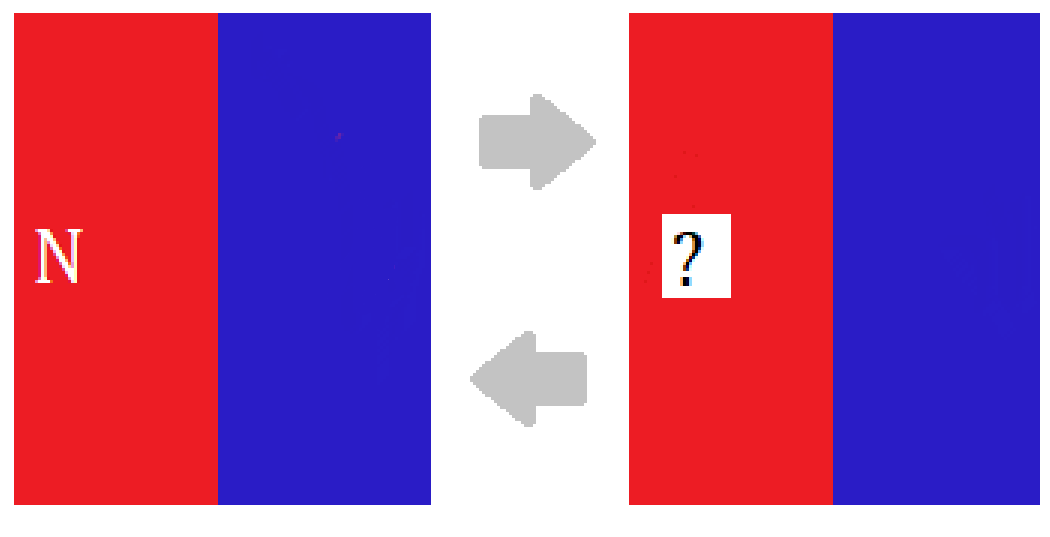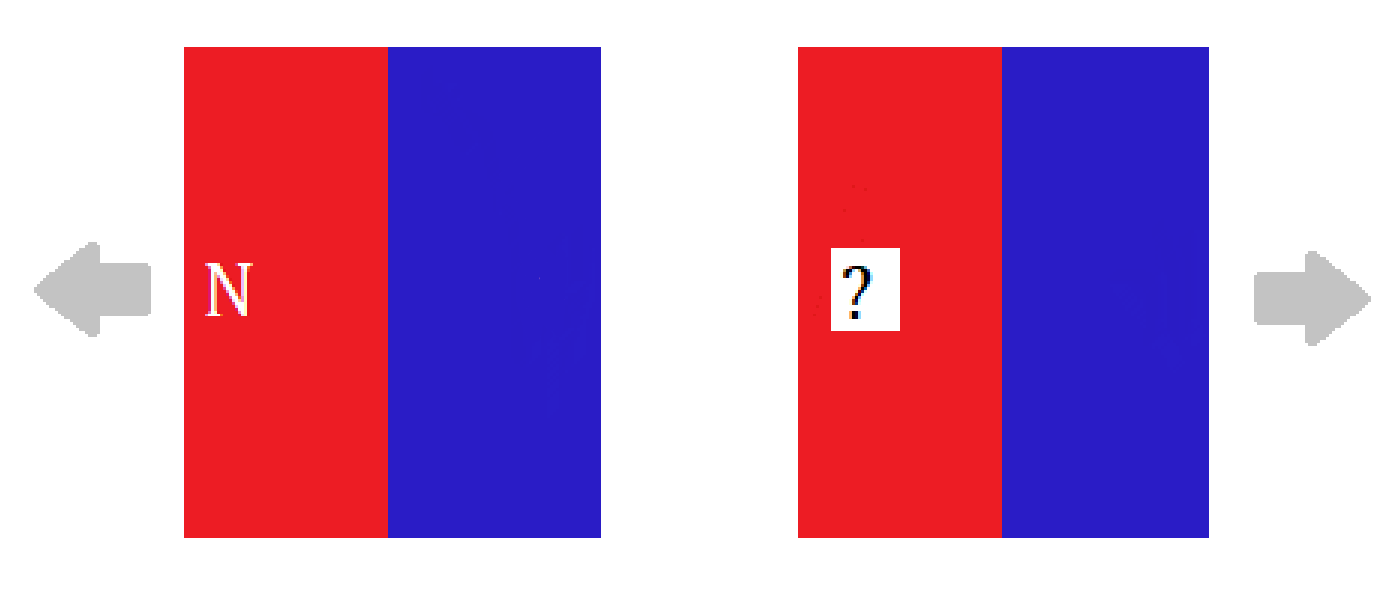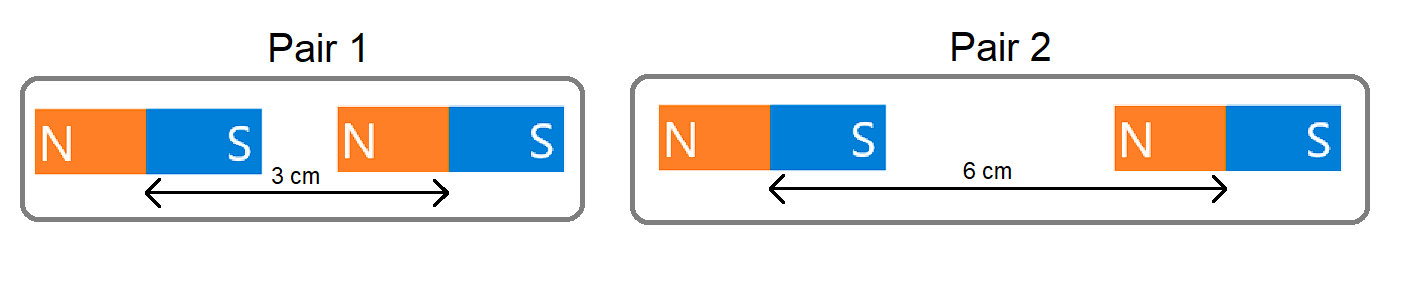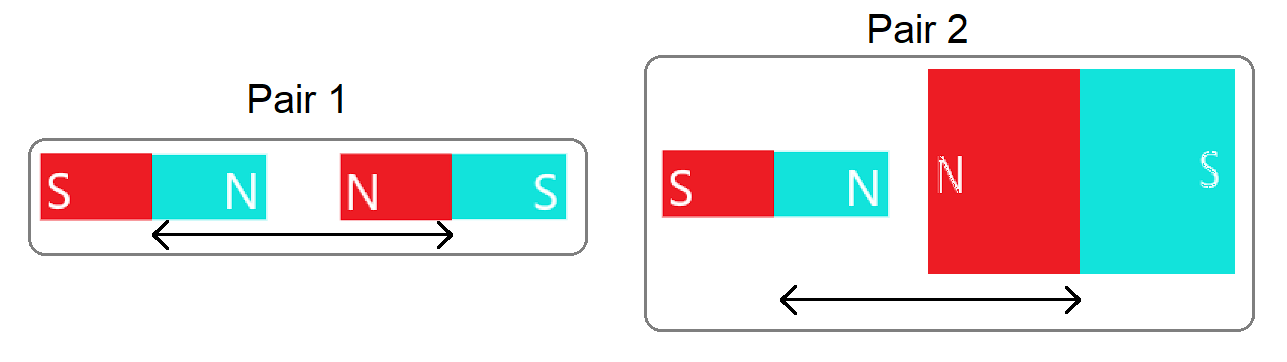# Learning About The Magnetic Force

This quiz contains questions related to the concept of magnets. Different types of questions related to the magnetic forces are asked in the quiz to clear the concept of magnetic forces for a student. The students are asked to find the direction of force when two magnets are placed near each other with different positions and also the effects of size and positions of magnets.

Start Quiz

Two magnets are placed as shown below.Will the magnets attract or repel each other?

Attract

Repel

Two magnets are placed as shown below.Will the magnets attract or repel each other?

Attract

Repel

Two magnets are placed as shown below.Will the magnets attract or repel each other?

Attract

Repel

Two magnets given below attract each other.Which pole would be present in the missing place?

N (North Pole)

S (South Pole)

Two magnets given below attract each other.Which pole would be present in the missing place?

N (North Pole)

S (South Pole)

Two magnets given below repel each other.Which pole would be present in the missing place?

N (North Pole)

S (South Pole)

The images below show two pairs of magnets. The magnets in different pairs do not affect each other. All the magnets shown are made of the same material.Think about the magnetic force between the magnets in each pair. Which of the following statements is true?

Pair 1 has greater magnetic force

Pair 2 has greater magnetic force

Both pairs have equal magnetic force

The images below show two pairs of magnets. The magnets in different pairs do not affect each other. All the magnets shown are made of the same material and are placed at equal distance, but some of them are different sizes and shapes.Think about the magnetic force between the magnets in each pair. Which of the following statements is true?

The magnetic force is weaker in Pair 1.

The magnetic force is weaker in Pair 2.

The strength of the magnetic force is the same in both pairs.

Quiz/Test Summary
Title: Learning About The Magnetic Force
Questions: 8
Contributed by: